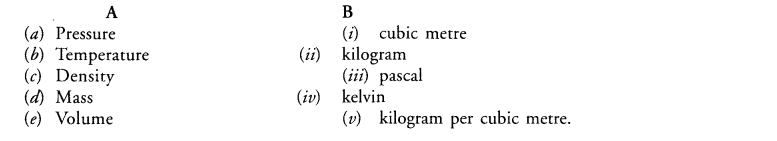# Match the physical quantities given in column A to their SI units given in Column B.`
Question:

Match the physical quantities given in column A to their SI units given in Column B.Solution:

$(\mathrm{a})-(\mathrm{iii}),(\mathrm{b})-(\mathrm{iv}),(\mathrm{c})-(\mathrm{v}),(\mathrm{d})-(\mathrm{ii}),(\mathrm{e})-(\mathrm{i}) .$